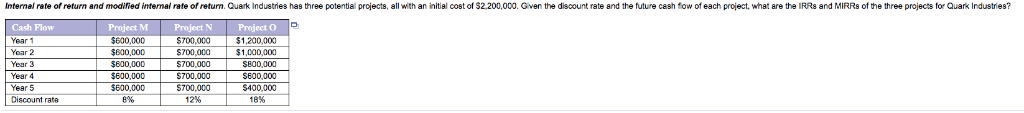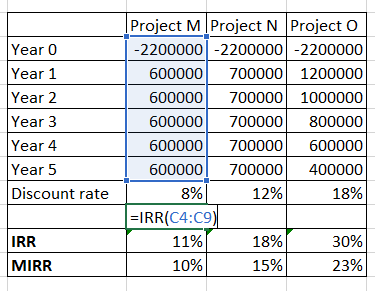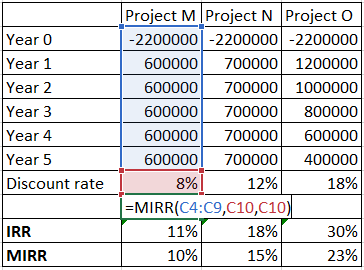# Internal rate of return and modified internal rate of return. Quark Industries has three potentia...Need help solving all parts thanks. Please show how answer was found.

Internal rate of return and modified internal rate of return. Quark Industries has three potential projects, all with an initial cost of \$2 200,000. Given the discount rate and the future cash fow of each project, what are the IRRs and MIRRs of the three projects for Quark Industries? M Cash Flow Year 1 Year 2 Year 3 Year 4 Year 5 Discount rate \$600,000 \$800,000 \$800,000 \$600,000 \$600,000 8% Project N 700,000 \$700,000 \$700,000 \$700,000 \$700,000 12% Project O \$1,200,000 \$1,000,000 SB00,000 S600,000 \$400,00 18%

IRR:

The interest rate which makes the net present worth of the project 0.

It can be calculated using IRR function in excel using the cash flows provided. Assume the Investment as a cash flow in Year 0 with a negative sign as the money goes out of your hand. In excel use syntax IRR (Values) where values signify the range of cash flowMIRR:

MIRR measures the return on a project and compares it with other potential project

It is calculated using MIRR function in excel where the syntax is MIRR (Values, finance rate, reinvest rate). Here the finance rate and reinvest rate refers to the discount rates of the project##### Add Answer of: Internal rate of return and modified internal rate of return. Quark Industries has three potentia...
More Homework Help Questions Additional questions in this topic.

• #### Analyze the following reactions in terms of constituent quarks and show that each type of quark...

Need Online Homework Help?